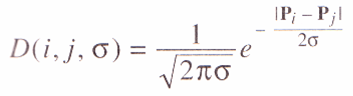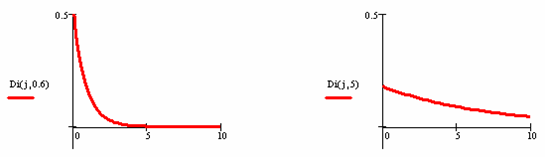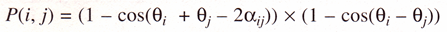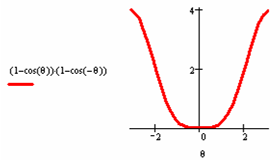# Computer Vision Demonstration Website

## Electronics and Computer ScienceUniversity of Southampton

### Symmetry Operator

The Symmetry Operator is designed to pick out the symmetrical properties of a shape. The images dont have to be classically symmetrical (e.g. squares or circles). The values of sigma determine the scope of the operator. Small values pick out very local symmetry whereas larger values pick out symmetry over greater distances. The operator uses the result of the Sobel operator as its input. This operator is extremely processor intensive.

### How it works

The operator forms an accumulator of points that are measures of symmetry between pixels in the image. During the processing, every pixel in the image must be compared to every other pixel. As such this is a very processor intensive operator.

Symmetry value of two points is based on:

• Distance weighting
• Phase weighting
• Edge magnitude at each point

#### Distance weighting

This determines how close together two points must be to contribute to the symmetrical value.where |Pi - Pj| is the distance between the two points and sigma is the locality of the operator:#### Phase weighting

The phase weighting depends on the relative direction of the edges between two points. The direction of the edges is calculated using:Where edges going in different directions have the greatest response:#### Total function

The total function considers the Distance Function, the Phase Function, and the Edge Magnitude (in Log form):

C(i,j,sigma) = D(i,j,sigma) * P(i,j) * log(1+E(i)) * log(1+E(j))

### Code

Image processing classes:

Demo framework:

The pages were designed and developed for educational purposes only, to demonstrate how computer vision techniques work. They are designed for no other purpose and neither the authors nor their institutions accept any liability concerning use of these pages.